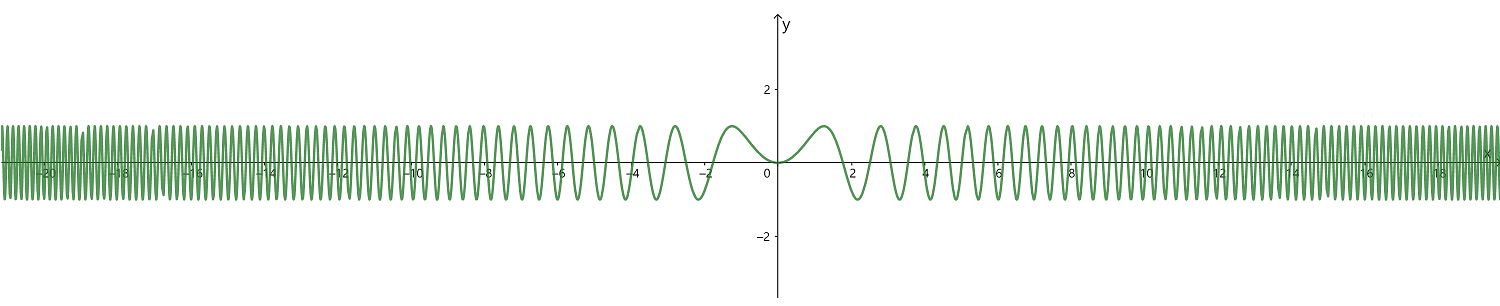安卓手机扫描二维码安装App﻿$\sin(x+T)^2$﻿ ﻿$=\sin x^2$﻿             (1)

﻿$x =$﻿ ﻿$?$﻿ ，得 ﻿$\sin T^2= 0$﻿ .

﻿$\sin(x+\sqrt{k\pi})^2$﻿﻿$=sin x^2$﻿           (2)

﻿$x=\sqrt{2}T$﻿ ，则﻿$x=\sqrt{2k\pi}$﻿ ，得

﻿$\sin(\sqrt{2}T +T)$﻿ ﻿$= \sin(\sqrt{2}T)^2$﻿

﻿$\sin[(\sqrt{2}+1)^2 k\pi]$﻿ ﻿$=\sin 2k\pi$﻿ ﻿$=0$﻿

﻿$(\sqrt{2}+1)^2 k\pi$﻿ ﻿$=l \pi$﻿ ﻿$(l \in N)$﻿

﻿$(\sqrt{2}+1)^2$﻿ ﻿$=\dfrac{l}{k}$﻿

﻿$(\sqrt{2}+1)^2$﻿ ﻿$=2+2\sqrt{2}$﻿ 是无理数，而 ﻿$\dfrac{l}{k}$﻿ 是有理数，矛盾，因此假设不成立，得证.苹果手机扫描二维码安装App